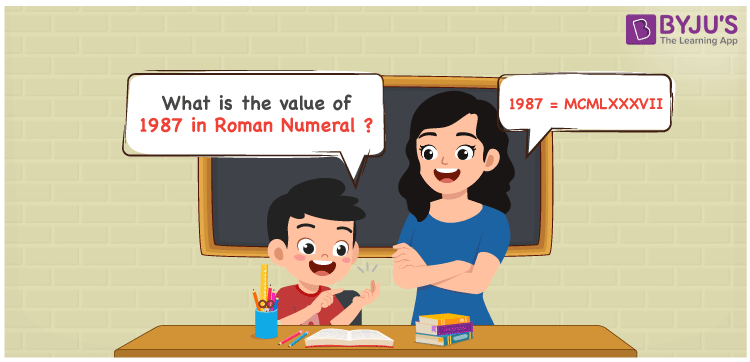# 1987 in Roman Numerals

1987 in Roman Numerals is MCMLXXXVII. The article on the roman numerals is deviced in such a way to improve the conceptual knowledge among the children. The step wise expansion of 1987 and its transformation to roman numerals can be learnt with ease here. Hence, 1987 can be written as MCMLXXXVII in roman numerals.

 Number Roman Numeral 1987 MCMLXXXVII## How to Write 1987 in Roman Numerals?

For an error free conversion of 1987 to roman numerals, refer to the steps provided below.

1987 = 1000 + (1000 – 100) + 50 + 10 + 10 + 10 + 5 + 1 + 1

1987 = M + (M – C) + L + X + X + X + V + I + I

1987 = MCMLXXXVII

## Video Lesson on Roman Numerals## Frequently Asked Questions on 1987 in Roman Numerals

Q1

### Write 1987 in Roman Numerals.

1987 in Roman Numerals is MCMLXXXVII.
Q2

### Calculate 2000 – 13.

We know that
2000 in Roman Numerals = MM
13 in Roman Numerals = XIII
As 2000 – 13 = 1987
So 2000 – 13 is 1987 which is written as MCMLXXXVII.
Q3

### How can 1987 be written in Roman Numerals?

1987 can be written in Roman Numerals by taking the ones, tens, hundreds and thousands value of the number.
Thousands = 1000 = M
Hundreds = 900 = CM
Tens = 80 = LXXX
Ones = 7 = VII
Number = 1000 + 900 + 80 + 7 = M + CM + LXXX + VII = MCMLXXXVII